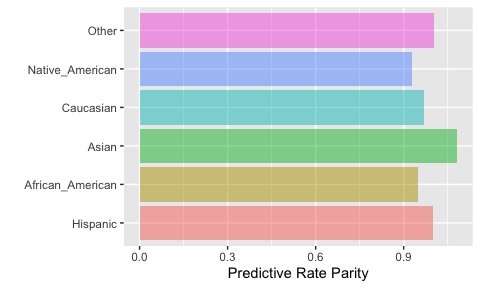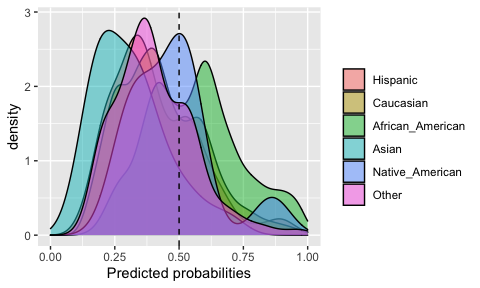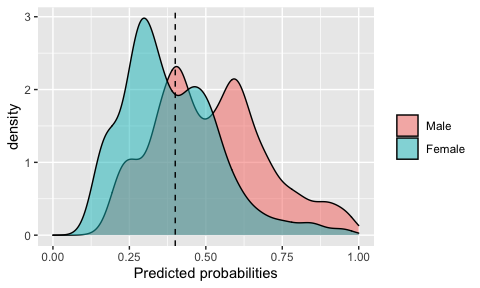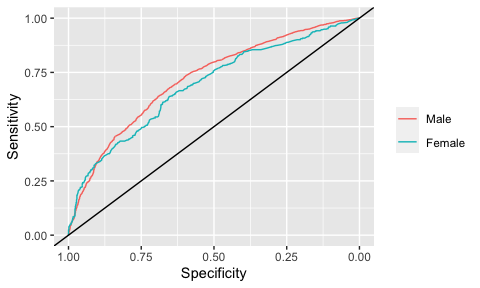A complete tutorial to the fairness R package

2020-11-19

This vignette provides a tutorial on using the fairness R package. A more detailed tutorial is also provided in this blogpost.

To date, a number of algorithmic group fairness metrics have been proposed. Demographic parity, proportional parity, equalized odds and predictive rate parity are the most commonly used metrics to evaluate fairness between groups in classification problems (supervised machine learning algorithms). Multiple other metrics have been proposed that use various metrics from the confusion matrix (e.g. false positive rate parity, false negative rate parity). The fairness R package provides a tool to easily calculate these metrics for given predicted probabilities or predicted classes between sensitive groups of populations. The package also provides visualizations that makes it easier to comprehend these metrics and biases between subgroups of the data.

Disclaimer! None of the implemented metrics are supposed to replace critical thinking about the problem in question.

Installation of the fairness R package

install.packages('fairness')
library(fairness)

Description of data

This package includes two datasets to study algorithmic fairness: compas and germancredit. In this tutorial, you will be able to use a simplified version of the landmark COMPAS dataset. You can read more about the dataset here. To load the dataset, all you need to do is:

data('compas')

The compas dataframe contains nine columns: The outcome is Two_yr_Recidivism, i.e. whether an individual will commit a crime in two years or not. Variables exist in the data about prior criminal record (Number_of_Priors and Misdemeanor) and basic features such as age, categorized (Age_Above_FourtyFive and Age_Below_TwentyFive), sex (Female) and ethnicity (ethnicity). You don’t really need to delve into the data much, we have already ran a prediction model using all variables to predict Two_yr_Recidivism and concatenated the predicted probabilities (probability) and predicted classes (predicted) to the data. You will be able to use the probability and predicted columns directly in your analysis.

However, please feel free to set up other prediction models (e.g. excluding sensitive group information, such as sex and ethnicity) and use your generated predicted probabilities or classes to assess group fairness.

As mentioned earlier, another dataset, germancredit is also included in this package. This dataset includes 20 potential predictor features and an outcome (target) column named BAD. Similarly to the compas dataset, this data also includes two pre-made columns named probability and predicted. Feel free to play wit this data as well! You can of course load it with:

data('germancredit')

Algorithmic group fairness metrics

The package currently includes nine fairness metrics and two other comparisons implemented. Many of these metrics are mutually exclusive - results from a given classification most often cannot be fair in terms of all evaluated group fairness metrics. Below, all these functions will be introduced in detail - for the sake of simplicity, we will use predicted probabilities for all these functions instead of predicted classes. Note that for all functions, in case of defining predicted probabilities, a cutoff needs to be defined in order to generate positive (1) or negative (0) predicted classes. Also, for all functions, a base group needs to be defined - all other groups will be compared to this subgroup in terms of fairness metrics.

An outlook on the confusion matrix

Most fairness metrics use various measures calculated from the confusion matrix. The confusion matrix is comprised of four distinct classes:

1. True positives (TP): True class positive and predicted positive (correct classification)
2. False positives (FP): True class negative and predicted positive (incorrect classification)
3. True negatives (TN): True class negative and predicted negative (correct classification)
4. False negatives (FN): True class positive and predicted negative (incorrect classification)

All fairness metrics are calculated using one or more of these measures. For a detailed overview of various measures and precise definitions, please click here, here or here.

Demographic parity

Demographic parity is achieved if the absolute number of positive predictions in the subgroups are close to each other. This measure does not take true class into consideration, only positive predictions.
Formula: (TP + FP)

dem_parity(data    = compas,
outcome = 'Two_yr_Recidivism',
group   = 'ethnicity',
probs   = 'probability',
preds_levels = c('no','yes'),
cutoff  = 0.5,
base    = 'Caucasian')

Proportional parity

Proportional parity is very similar to Demographic parity. Proportional parity is achieved if the proportion of positive predictions in the subgroups are close to each other. This measure does not take true class into consideration, only positive predictions.
Formula: (TP + FP) / (TP + FP + TN + FN)

prop_parity(data    = compas,
outcome = 'Two_yr_Recidivism',
group   = 'ethnicity',
probs   = 'probability',
preds_levels = c('no','yes'),
cutoff  = 0.5,
base    = 'Caucasian')

All the rest of the functions take the true class into consideration.

Equalized odds

Equalized odds are achieved if the sensitivities (true positives divided by all positives) in the subgroups are close to each other.
Formula: TP / (TP + FN)

equal_odds(data    = compas,
outcome = 'Two_yr_Recidivism',
group   = 'ethnicity',
probs   = 'probability',
preds_levels = c('no','yes'),
cutoff = 0.5,
base   = 'African_American')

Predictive rate parity

Predictive rate parity is achieved if the precisions or positive predictive values (true positives divided by all predicted positive) in the subgroups are close to each other.
Formula: TP / (TP + FP)

pred_rate_parity(data    = compas,
outcome = 'Two_yr_Recidivism',
group   = 'ethnicity',
probs   = 'probability',
preds_levels = c('no','yes'),
cutoff = 0.5,
base   = 'African_American')

Accuracy parity

Accuracy parity is achieved if the accuracies (all accurately classified divided by all predictions) in the subgroups are close to each other.
Formula: (TP + TN) / (TP + FP + TN + FN)

acc_parity(data    = compas,
outcome = 'Two_yr_Recidivism',
group   = 'ethnicity',
probs   = 'probability',
preds   = NULL,
preds_levels = c('no','yes'),
cutoff = 0.5,
base   = 'African_American')

False negative rate parity

False negative rate parity is achieved if the false negative rates (division of false negatives with all positives) in the subgroups are close to each other.
Formula: FN / (TP + FN)

fnr_parity(data    = compas,
outcome = 'Two_yr_Recidivism',
group   = 'ethnicity',
probs   = 'probability',
preds_levels = c('no','yes'),
cutoff = 0.5,
base   = 'African_American')

False positive rate parity

False positive rate parity is achieved if the false positive rates (division of false positives with all negatives) in the subgroups are close to each other.
Formula: FP / (TN + FP)

fpr_parity(data    = compas,
outcome = 'Two_yr_Recidivism',
group   = 'ethnicity',
probs   = 'probability',
preds_levels = c('no','yes'),
cutoff = 0.5,
base   = 'African_American')

Negative predictive value parity

Negative predictive value parity is achieved if the negative predictive values (division of true negatives with all predicted negatives) in the subgroups are close to each other. This function can be considered the ‘inverse’ of Predictive rate parity.
Formula: TN / (TN + FN)

npv_parity(data    = compas,
outcome = 'Two_yr_Recidivism',
group   = 'ethnicity',
probs   = 'probability',
preds_levels = c('no','yes'),
cutoff = 0.5,
base   = 'African_American')

Specificity parity

Specificity parity is achieved if the specificities (division of true negatives with all negatives) in the subgroups are close to each other. This function can be considered the ‘inverse’ of Equalized odds.
Formula: TN / (TN + FP)

spec_parity(data    = compas,
outcome = 'Two_yr_Recidivism',
group   = 'ethnicity',
probs   = 'probability',
preds_levels = c('no','yes'),
cutoff = 0.5,
base   = 'African_American')

Two additional comparisons are implemented, namely ROC AUC and Matthews correlation coefficient comparisons.

ROC AUC comparison

This function calculates ROC AUC and visualizes ROC curves for all subgroups. Note that probabilities must be defined for this function. Also, as ROC evaluates all possible cutoffs, the cutoff argument is excluded from this function.

roc_parity(data    = compas,
outcome = 'Two_yr_Recidivism',
group   = 'ethnicity',
probs   = 'probability',
preds_levels = c('no','yes'),
base = 'African_American')

Matthews correlation coefficient comparison

The Matthews correlation coefficient takes all 4 classes of the confusion matrix into consideration. According to some, it is the single most powerful metric in binary classification problems, especially for data with class imbalances.
Formula: (TP×TN-FP×FN)/√((TP+FP)×(TP+FN)×(TN+FP)×(TN+FN))

mcc_parity(data    = compas,
outcome = 'Two_yr_Recidivism',
group   = 'ethnicity',
probs   = 'probability',
preds_levels = c('no','yes'),
cutoff = 0.5,
base   = 'African_American')

Output and visualizations

All functions output results and matching barcharts that provide visual cues about the parity metrics for the defined sensitive subgroups. For instance, let’s look at predictive rate parity with ethnicity being set as the sensitive group and considering Caucasians as the ‘base’ group:

output$Metric #> Caucasian African_American Asian Hispanic #> Precision 0.6967156 0.6828704 0.7777778 0.7194444 #> Predictive Rate Parity 1.0000000 0.9801279 1.1163490 1.0326229 #> Group size 2103.0000000 3175.0000000 31.0000000 509.0000000 #> Native_American Other #> Precision 0.6666667 0.7209302 #> Predictive Rate Parity 0.9568706 1.0347554 #> Group size 11.0000000 343.0000000 In the upper row, the raw precision values are shown for all ethnicities, and in the row below, the relative precisions compared to Caucasians (1) are shown. Note that in case an other ethnic group is set as the base group (e.g. Hispanic), the raw precision values do not change, only the relative metrics: output$Metric
#>                           Hispanic    Caucasian African_American      Asian
#> Precision                0.7194444    0.6967156        0.6828704  0.7777778
#> Predictive Rate Parity   1.0000000    0.9684078        0.9491634  1.0810811
#> Group size             509.0000000 2103.0000000     3175.0000000 31.0000000
#>                        Native_American       Other
#> Precision                    0.6666667   0.7209302
#> Predictive Rate Parity       0.9266409   1.0020652
#> Group size                  11.0000000 343.0000000

A standard output is a barchart that shows the relative metrics for all subgroups. For the previous case (when Hispanic is defined as the base group), this plot would look like this:

output$Metric_plotWhen probabilities are defined, an extra density plot will be output with the distributions of probabilities of all subgroups and the user-defined cutoff: output$Probability_plotAnother example would be comparing males vs. females in terms of recidivism prediction and defining a 0.4 cutoff:

output$Probability_plotThe function related to ROC AUC comparisons will output ROC curves for each subgroups. Let’s look at the plot, also comparing males vs. females: output$ROCAUC_plotClosing words

You have read through the fairness R package tutorial and by now, you have a solid grip on algorithmic group fairness metrics. We hope that you will be able to use this R package in your data analysis! Please let us know if you have any issues here - fairness GitHub - or contact the authors if you have any feedback!

– authors of the fairness R package